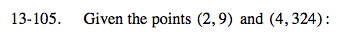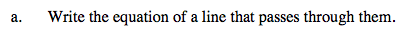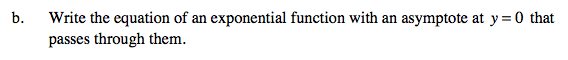### Home > A2C > Chapter 13 > Lesson 13.2.1 > Problem13-105

13-105.$\text{Use }m=\frac{y_2-y_1}{x_2-x_1}\text{ to find the slope of the line.}$

Using the slope-intercept form of a line and a given point, find b.

$y=\frac{315}{2}x-306$Use y = kmx and substitute the values for x and y from both points, creating two equations.

Now divide the equations and solve for m.

$\frac{324}{9}=\frac{km^4}{km^2}$

Now that you know m, find the value of k.

y = 0.25(6)x